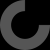# Report as inappropriate

Here's the top bit of my CURA profile settings...
I only use PET-G, and have had no issues with retraction or jams using this profile...

[profile]
layer_height = 0.2
wall_thickness = 0.8
retraction_enable = True
solid_layer_thickness = 0.8
fill_density = 20
nozzle_size = 0.4
print_speed = 40
print_temperature = 250
print_temperature2 = 0
print_temperature3 = 0
print_temperature4 = 0
print_temperature5 = 0
print_bed_temperature = 60
support = Touching buildplate
support_dual_extrusion = Both
wipe_tower = False
wipe_tower_volume = 15
ooze_shield = False
filament_diameter = 1.75
filament_diameter2 = 0
filament_diameter3 = 0
filament_diameter4 = 0
filament_diameter5 = 0
filament_flow = 100.0
retraction_speed = 25
retraction_amount = 2
retraction_dual_amount = 16.5
retraction_min_travel = 1.5
retraction_combing = All
retraction_minimal_extrusion = 0.02
retraction_hop = 0.0
bottom_thickness = 0.3
layer0_width_factor = 125
object_sink = 0.0
overlap_dual = 0.15
travel_speed = 90
bottom_layer_speed = 15
infill_speed = 0.0
solidarea_speed = 0.0
inset0_speed = 20
insetx_speed = 0.0
cool_min_layer_time = 15
fan_enabled = True
skirt_line_count = 1
skirt_gap = 3.0
skirt_minimal_length = 150.0
fan_full_height = 0.5
fan_speed = 100
fan_speed_max = 100
cool_min_feedrate = 10
solid_top = True
solid_bottom = True
fill_overlap = 15
perimeter_before_infill = False
support_type = Lines
support_angle = 60
support_fill_rate = 15
support_xy_distance = 0.7
support_z_distance = 0.15
spiralize = False
simple_mode = False
brim_line_count = 40
raft_margin = 5.0
raft_line_spacing = 3.0
raft_base_thickness = 0.3
raft_base_linewidth = 1.0
raft_interface_thickness = 0.27
raft_interface_linewidth = 0.4
raft_airgap_all = 0.0
raft_airgap = 0.22
raft_surface_layers = 2
raft_surface_thickness = 0.27
raft_surface_linewidth = 0.4
fix_horrible_union_all_type_a = True
fix_horrible_union_all_type_b = False
fix_horrible_use_open_bits = False
fix_horrible_extensive_stitching = False
plugin_config =
object_center_x = -1
object_center_y = -1